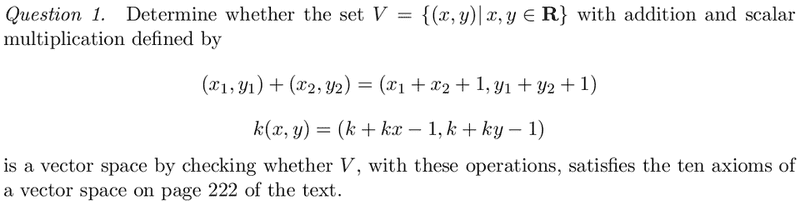# Determining vector spaces

dcramps

## Homework Statement## Homework Equations

The 10 axioms:
1. If u and v are objects in V, then u + v is in V
2. u + v = v + u
3. u + (v+w) = (u+v)+w
4. There is an object 0 in V, called a zero factor for V, such that 0+u = u+0 = u for all u in V
5. For each u in V, there is an object -u in V, called a negative of u, such that u+(-u)=(-u)+u = 0
6. If k is any scalar and u is any object in V, then ku is in V
7. k(u + v) = ku + kv
8. (k + m)u = ku + mu
9. k(mu) = (km)(u)
10. 1u = u

## The Attempt at a Solution

First of all, are u, v, and w just (x_{1},y_{1}), (x_{2},y_{2}), (x_{3},y_{3}) and so on? If so, am I going about this the right way?

Axiom 1. Since x,y$$\in$$R, then any (x,y)$$\in$$ thus the addition would hold. (Not really sure how to explain this one well)
Axiom 2. $$(x_{1},y_{1})+(x_{2},y_{2}) = (x_{1}+x_{2}+_{1},y_{1}+y_{2}+_{1}) = (x_{2},y_{2})+(x_{1},y_{1}) = (x_{2}+x_{1}+_{1},y_{2}+y_{1}+_{1})$$
Axiom 3.
$$u=(x_{1},y_{1})$$
$$v=(x_{2},y_{2})$$
$$w=(x_{3},y_{3})$$

$$u+(v+w) = (x_{1},y_{1})+(x_{2}+x_{3}+1,y_{2}+y_{3}+1) = (x_{1}+(x_{2}+x_{3}+1)+1,y_{1}+(y_{2}+y_{3}+1)+1) = (x_{1}+x_{2}+x_{3}+_{2},y_{1}+y_{2}+y_{3}+2)$$
$$(u+v)+w = (x_{1}+x_{2}+1,y_{1}+y_{2}+1) + (x_{3},y_{3}) = ((x_{1}+x_{2}+_{1})+x_{3}+1,(y_{1}+y_{2}+1)+y_{3}+1) = (x_{1}+x_{2}+x_{3}+_{2},y_{1}+y_{2}+y_{3}+2)$$

and so on for the rest...

Is this the correct approach? It seems that it is to me, but I may be way off here.

Last edited:

Mentor

## Homework Statement## Homework Equations

The 10 axioms:
1. If u and v are objects in V, then u + v is in V
2. u + v = v + u
3. u + (v+w) = (u+v)+w
4. There is an object 0 in V, called a zero factor for V, such that 0+u = u+0 = u for all u in V
That would be zero vector, not factor.
5. For each u in V, there is an object -u in V, called a negative of u, such that u+(-u)=(-u)+u = 0
6. If k is any scalar and u is any object in V, then ku is in V
7. k(u + v) = ku + kv
8. (k + m)u = ku + mu
9. k(mu) = (km)(u)
10. 1u = u

## The Attempt at a Solution

First of all, are u, v, and w just (x_{1},y_{1}), (x_{2},y_{2}), (x_{3},y_{3}) and so on? If so, am I going about this the right way?
Yes, u, v, and w and just ordered pairs.
Axiom 1. Since x,y$$\in$$R, then any (x,y)$$\in$$ thus the addition would hold.
You left something out. ... then any (x, y) $\in$ V, so V is closed under addition.
(Not really sure how to explain this one well)
Axiom 2. $$(x_{1},y_{1})+(x_{2},y_{2}) = (x_{1}+x_{2}+_{1},y_{1}+y_{2}+_{1}) = (x_{2},y_{2})+(x_{1},y_{1}) = (x_{2}+x_{1}+_{1},y_{2}+y_{1}+_{1})$$
Axiom 3.
$$u=(x_{1},y_{1})$$
$$v=(x_{2},y_{2})$$
$$w=(x_{3},y_{3})$$

$$u+(v+w) = (x_{1},y_{1})+(x_{2}+x_{3}+1,y_{2}+y_{3}+1) = (x_{1}+(x_{2}+x_{3}+1)+1,y_{1}+(y_{2}+y_{3}+1)+1) = (x_{1}+x_{2}+x_{3}+_{2},y_{1}+y_{2}+y_{3}+2)$$
$$(u+v)+w = (x_{1}+x_{2}+1,y_{1}+y_{2}+1) + (x_{3},y_{3}) = ((x_{1}+x_{2}+_{1})+x_{3}+1,(y_{1}+y_{2}+1)+y_{3}+1) = (x_{1}+x_{2}+x_{3}+_{2},y_{1}+y_{2}+y_{3}+2)$$

and so on for the rest...

Is this the correct approach? It seems that it is to me, but I may be way off here.
Yes, this is the right approach. I have my suspicions, though, that V is not a vector space.

dcramps
Thanks for the tip and clarification :]

I think you are correct, unless I made a mistake. Using this approach I found that all axioms were satisfied except axiom 5. Thoughts on this?

Mentor
Axiom 4 is also violated. There might be others, but I didn't check all of them.

Staff Emeritus
Homework Helper
Thanks for the tip and clarification :]

I think you are correct, unless I made a mistake. Using this approach I found that all axioms were satisfied except axiom 5. Thoughts on this?

Axiom 4 is also violated. There might be others, but I didn't check all of them.
Both axioms hold, actually. The zero vector would be 0=(-1,-1), and the additive inverse would be -(x,y) = (-x-2,-y-2). I would have thought one of axioms 7-10 would fail, but I think they all work out.

Mentor
Live and learn. Thanks, Vela.